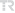## Kirinim teorisindeki bir takim iki boyutlu problemlerin çözümlenebilmesi için efektif bir metot An effective method for solving one class of integral equations in two-dimensional problems of diffraction theory

Journal of the Faculty of Engineering and Architecture of Gazi University, vol.37, no.2, pp.1103-1110, 2022 (SCI-Expanded)• Publication Type: Article / Article
• Volume: 37 Issue: 2
• Publication Date: 2022
• Doi Number: 10.17341/gazimmfd.869834
• Journal Name:
• Journal Indexes: Science Citation Index Expanded (SCI-EXPANDED), Scopus, Academic Search Premier, Art Source, Compendex, TR DİZİN (ULAKBİM)
• Page Numbers: pp.1103-1110
• Keywords: Electromagnetics, diffraction theory, perfect electric conductor, IMPEDANCE STRIP, HALF-PLANE, SCATTERING, WAVE
• Gazi University Affiliated: Yes

#### Abstract

© 2022 Gazi Universitesi Muhendislik-Mimarlik. All rights reserved.In nowadays, two dimensional integral equations are frequently used in various different engineering and scientific studies. In solving these equations using numerical and analytical methods along wide frequency spectrums; the electrical dimensions of the objects and the complexity of their geometric structures are considered as critical computational aspects.This article offers an effective solution to solve some integral equations. Various problems of the theory of diffraction realized by perfectly conductive metal plates are reduced to these integral equations. Thus, a method for solving integral equations is proposed to understand arbitrary values of frequency parameters and strip sizes. Diffraction theory, defines the diffraction processes that occur when waves such as in the form of light, encounter different obstacles in multiples of their wavelengths with dimensions. A certain bending and deflection of waves around these barriers is characterized by diffraction theory. In dealing with diffraction theory, advanced numerical and matrix-based solutions such as the moment method and the T-matrix method are used. On the other hand, the integralbased solutions proposed in this study are used effectively. The integral-based solution obtained from this study is simulated according to the different medium permeability which is calculated with the electrical dimension varying depending on the frequency of the wave, and a graphical representation is provided. It is seen that the normalized current value at the ends of the strip is maximum for two different cases where the medium permeability is 20 and 30.